The aim of this project was to create a system on roof which can collect water during rain.

The Idea is based on some Algeas on rooftops which can grow during the seasons in bowl Like shape in order to collect water inside them and connect them to the mechanical pipes of the pavilion. The shapes of the algeas can generate in each iteration based on wind , temperature and rainfalls.
In order to visualize the shape I decided to use magnetic field with some agents and move them on some random vectors in Z direction so the shape can change each time of the iteration based on rule of magnetic fields.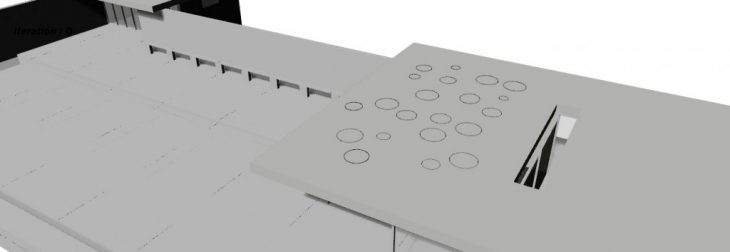1. generate random cirlces on roof with different Radiuses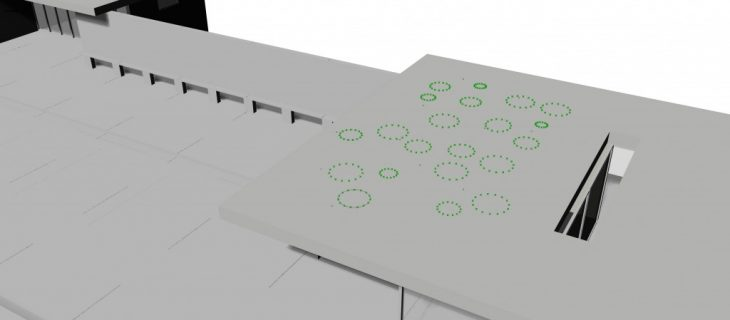2. divide each curves with several points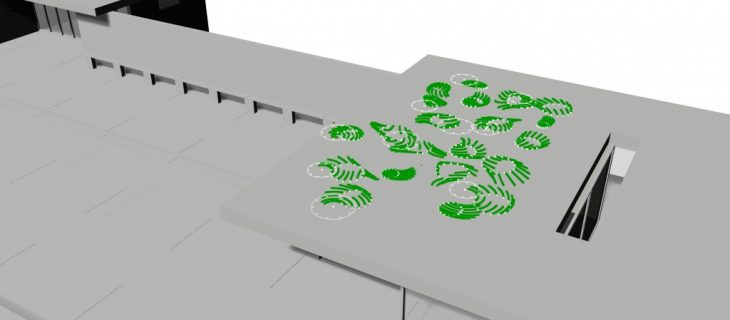3.connect the point to Anemone input
4. generate several points on roof
5. give each of the points point charge and point force Rule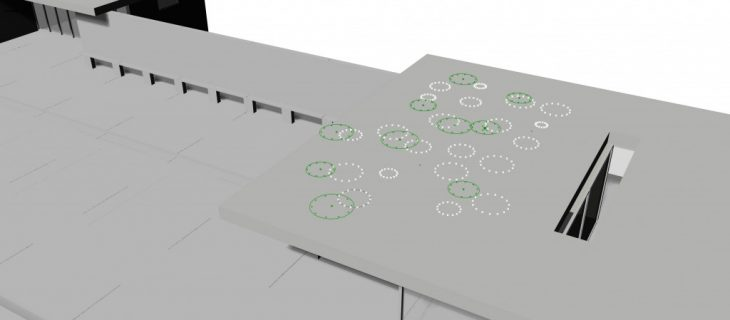6. make the divided points move on magnetic field
( iteration 20)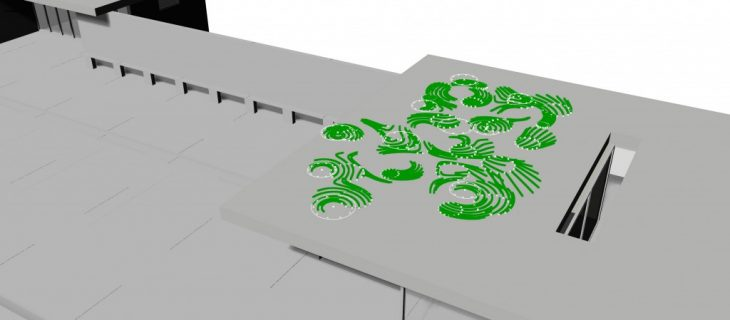7. Moving points in the magnetic field based on Tensor field and Y vector ( iteration 80 )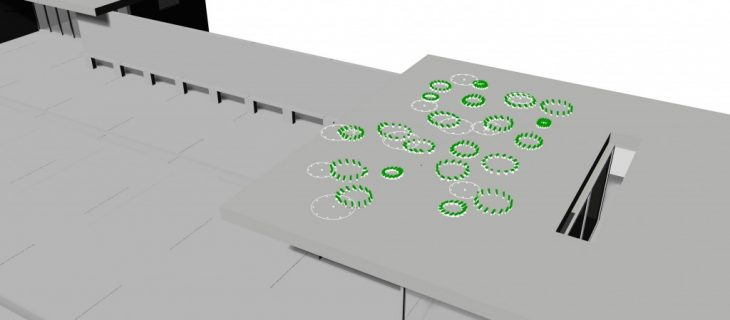8.Moving each iteration on Z axis (Iteration 10)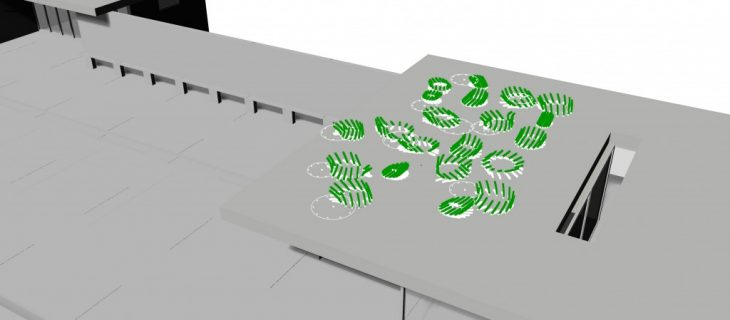9.Moving each iteration on Z axis (Iteration 40)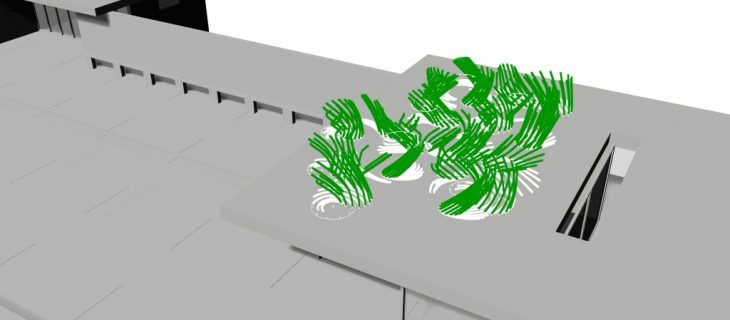10.Moving each iteration on Z axis (Iteration 90)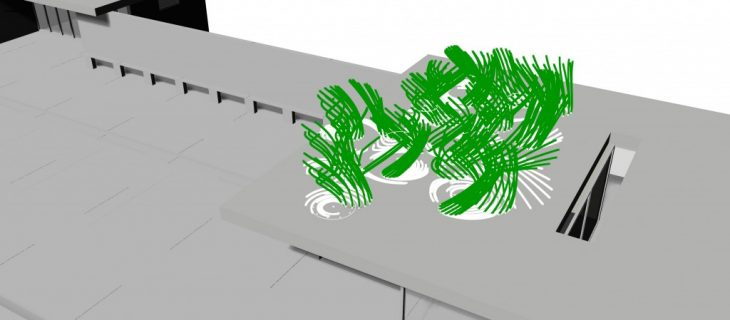11.Moving each iteration on Z axis (Iteration 120)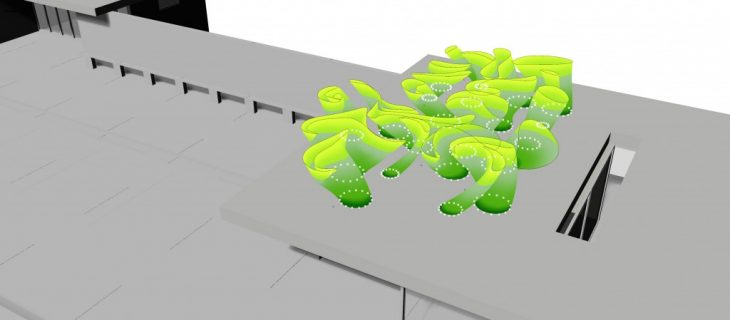12. Mesh Loft the points (Iteration 120)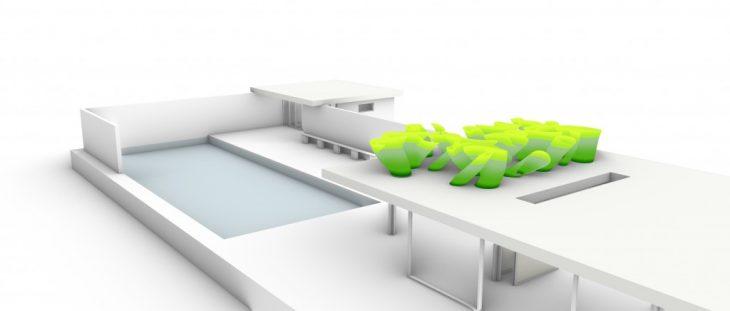Final Result